### RCHART, User Guide

Copyright 2005, J4L Components (http://www.java4less.com)
Go bak to contents

### Barchart configuration / parameters

#### Bar and column chart

If you want to create barchart you must use SERIE_TYPE_*=BAR (or BarPlotter class). The following is a complete example of a barchart with 2 series and negative values:

 Parameters Description Equivalent java class/property TITLECHART=Sales 2002 XLABEL=Month YLABEL=Million \$ XSCALE_MIN=0 XSCALE_MAX=5.5 YSCALE_MIN=-15 BIG_TICK_INTERVALX=1 BIG_TICK_INTERVALY=10 XAXIS_LABELS=June|July|Aug.|Sept.|Oct.|Nov.|Dec. CERO_XAXIS=LINE YAXIS_INTEGER=TRUE SERIE_1=Products SERIE_2=Services SERIE_TYPE_1=BAR SERIE_TYPE_2=BAR SERIE_FONT_1=Arial|PLAIN|8 SERIE_FONT_2=Arial|PLAIN|8 SERIE_DATA_1=12|43|50|45|30 SERIE_DATA_2=-10|41|48|39|36 SERIE_BORDER_TYPE_1=RAISED SERIE_BORDER_TYPE_2=RAISED SERIE_BAR_STYLE_1=0xff00 SERIE_BAR_STYLE_2=0xff BARCHART_BARSPACE=1 LEFT_MARGIN=0.15 CHART_FILL=0xffcc00 SERIE_NEGATIVE_STYLE_2=RED Title and labels X scale is 0-5.5 Y scale starts at -15 X axis labels Draw line at possition 0 of Y Axis Do not use decimal in the scale Select barchart type Font of the values above the bars Values of the bars Bars should have "raised" effect Color of the bars Background color of the chart Negative bars have red color new Title("Sales 2002"); chart.XLabel=new HAxisLabel("Month",Color,font); chart.YLabel=new VAxisLabel("Million \$",Color,font); chart.XAxis.scale.min=0; chart.XAxis.scale.max=5.5; chart.YAxis.scale.min=-15; chart.XAxis.bigTickInterval=1; chart.XAxis.tickLabels{"June",...}; chart.XAxis.ceroAxis=Axis.CEROAXIS_LINE; chart.YAxis.IntegerScale=true; legend.addItem("Products",style); serie1=new BarDataSerie(values,fillStyle); serie1.valueFont=new Font(...); see values in BarDataSerie constructor serie.borderType=BarDataSerie.BORDER_RAISED; see style in BarDataSerie constructor barPlotter.interBarSpace=1; chart.leftMargin=0.15; serie2.negativeStyle=new FillStyle(Color.red);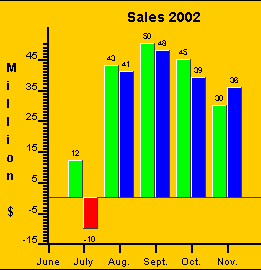By changing the following parameters you can convert it to a horizontal barchart:

 Parameters Description Equivalent java class/property BARCHART_VERTICAL=FALSE XSCALE_MIN=-15 BIG_TICK_INTERVALY=1 YAXIS_LABELS=June|July|Aug.|Sept.|Oct.|Nov.|Dec. XAXIS_START_WITH_BIG_TICK=TRUE XAXIS_INTEGER=TRUE Horizontal bars Definition of the scales/axis Only big ticks (no small ticks) Labels for the scale There should be a big tick at position 0 barPlotter.verticalBar chart.XAxis.scale.min=-15; chart.YAxis.bigTickIntervalList=1; chart.YAxis.tickLabels{"June",...}; chart.XAxis.startWithBigTick=true; chart.XAxis.IntegerScale=true;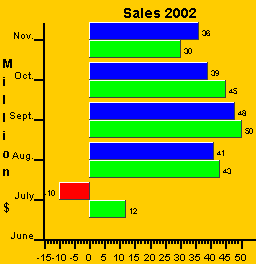Bars can also be forced to start at a fixed possition instead of the default, which is 0.

 Parameters Description Equivalent java class/property BARCHART_BASE=5 Starting possition of bars barPlotter.barsBase=5;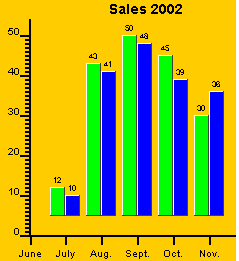You can also assign a different color to each bar in your series.

 Parameters Description Equivalent java class/property SERIE_BARS_COLORS_1=RED|BLUE|BLACK|YELLOW|CYAN Colors of the bars serie1.barStyles=styles;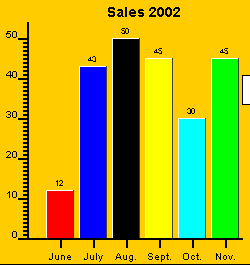Each bar can also start at a different possition by means of the SERIE_BARS_START_ parameter .

 Parameters Description Equivalent java class/property SERIE_DATA_1=12|43|50|45|30|45 SERIE_BARS_START_1=5|0|15|10|5 End possition of the bars. Start possition of the bars. see values in BarDataSerie constructor serie1.addBarsBase (startValues);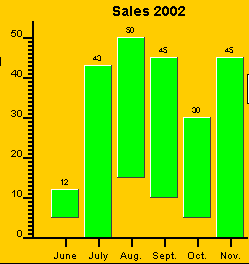The following parameters will allow you to control the width:

The main parameter is BARCHART_BARWIDTH

 Parameters Description Equivalent java class/property BARCHART_BARWIDTH=4 Bar's width barPlotter.barWidth=4;

this parameter has priority over the other parameters.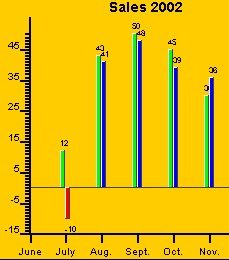If you don't want to specify the width of the bars you can also specify the distance between bars and the distance between groups of bars:

 Parameters Description Equivalent java class/property BARCHART_BARSPACE=4 space between bars barPlotter.interBarSpace=4;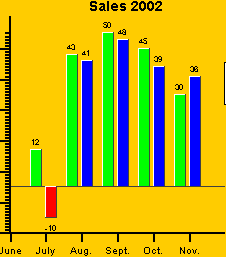if you have only 2 bars you can end up with the right bar overlapping the legend: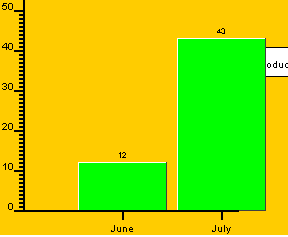you can solve this problem by adding 0.5 to the maximum value of the scale. In the example:

 Parameters Description Equivalent java class/property XSCALE_MAX=2.5 Make the X axis a bit longer then required so that it looks better. chart.XAxis.scale.max=2.5;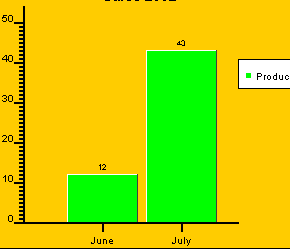There is avery common error which result is getting the following chart: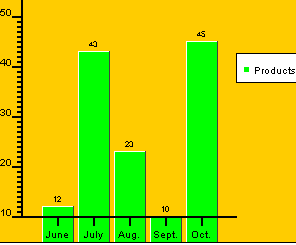which can be solved by setting the correct value for the Y scale:

 Parameters Description Equivalent java class/property YSCALE_MIN=0 Starting point of the Y scale. chart.YAxis.scale.min=0;#### Stackbar chart

If you want the values of the series to be added (stackbar) then you must set BARCHART_CUMULATIVE=TRUE like in the following example:

 Parameters Description Equivalent java class/property BARCHART_CUMULATIVE=TRUE SERIE_DATA_1=12|43|50|45|30 SERIE_DATA_2=10|41|48|39|36 SERIE_TYPE_1=BAR SERIE_TYPE_2=BAR Activate stackbar chart Values Barchart barPlotter.cumulative see values in BarDataSerie constructor#### Eventchart

Eventcharts have the following properties:

1. All values in the serie are aligned in the same line (SERIE_DATAX_ parameter).
2. Each value is made of a starting point and an ending point (SERIE_BARS_START_ and SERIE_DATA_)

In this way the chart can represent length of activities.

 Parameters Description Equivalent java class/property SERIE_BARS_START_1=0|15|30 SERIE_DATA_1=5|27|44 SERIE_DATAX_1=0|0|0 SERIE_BARS_START_2=5|10|20 SERIE_DATA_2=7|15|24 SERIE_DATAX_2=1|1|1 BARCHART_VERTICAL=false BARCHART_EVENTCHART=true Starting possitions of bars (serie 1) Ending possitions of bars (serie 1) bars centered on first tick (Act A) Starting possitions of bars (serie 2) Ending possitions of bars (serie 2) bars centered on second tick (Act B) Horizontal bars event chart (bars centered on tick) serie1.addBarsBase (startValues); see values in BarDataSerie constructor serie1.setDatax(...); barPlotter.eventChart=true;#### Barchart 3D

If you want to create 3D barchart you must use SERIE_TYPE_*=BAR3D (or BarPlotter3D class). The following is a complete example:

 Parameters Description Equivalent java class/property TITLECHART=Sales 1999 XLABEL=Month YLABEL=Million \$ XSCALE_MIN=0 YSCALE_MIN=-15 XSCALE_MAX=5.5 TICK_INTERVALY=5 BIG_TICK_INTERVALX=1 BIG_TICK_INTERVALY=1 XAXIS_GRID=0.2|0xc0c0c0|DASHED XAXIS_GRID=0.2|0xc0c0c0|DASHED XAXIS_LABELS=June|July|Aug.|Sept.|Oct.|Nov.|Dec. YAXIS_INTEGER=TRUE LEGEND_FILL=WHITE LEGEND_BORDER=0.2|0x0|NORMAL SERIE_1=Products SERIE_2=Services SERIE_TYPE_1=BAR3D SERIE_TYPE_2=BAR3D SERIE_DATA_1=12|43|50|45|30 SERIE_DATA_2=-10|41|48|39|36 SERIE_BAR_STYLE_2=0xff00 BARCHART_BARSPACE=1 BARCHART3D_DEPTH=15 BARCHART3D_FULL_DEPTH=TRUE BARCHART3D_BACK=WHITE CHART_BORDER=0.2|0x0|NORMAL CHART_FILL=LIGHTGRAY BACK_IMAGE=back13.gif Title and labels X scale is 0-5.5 Y scale starts at -15 Activate grid X axis labels Do not use decimal in the scale Select barchart 3D type Values of the bars Color of the bar Length of Z axis Background image new Title("Sales 1999"); chart.XLabel=new HAxisLabel("Month",Color,font); chart.YLabel=new VAxisLabel("Million \$",Color,font); chart.XAxis.scale.min=0; chart.YAxis.scale.min=-15; chart.XAxis.scale.max=5.5; chart.YAxis.scaleTickInterval=5; chart.XAxis.bigTickInterval=1; chart.XAxis.gridStyle=new LineStyle(...); chart.XAxis.tickLabels{"June",...}; chart.YAxis.IntegerScale=true; legend.background=new FillStyle(Color.white); legend.addItem("Products",style); serie1=new BarDataSerie(values,fillStyle); see values in BarDataSerie constructor see style in BarDataSerie constructor barPlotter3D.interBarSpace=1; barPlotter3D.depth=15; barPlotter3D.fullDepth=true; barPlotter3D.back=new FillStyle(Color.white); chart.backImage=image;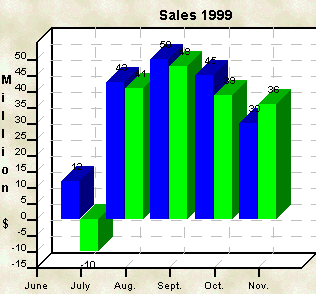All parameters are the same as in the 2D barcharts. The following parameter are 3D barchart specific:

the following parameter controls the depth of the bars:

 Parameters Description Equivalent java class/property BARCHART3D_FULL_DEPTH=false Bars should not be as deep as the Z axis. In this case the depth of the bars will be the length of the Z axis / 2. barPlotter3D.fullDepth=false;You can specify the length of the Z axis with:

 Parameters Description Equivalent java class/property BARCHART3D_DEPTH=5 Change length of the Z axis (pixels) barPlotter3D.depth=5;